# Quiz 9: Multicriteria Decision Making

Prepare the goal programming model by following the steps as shown below: Step 1: Formulate the goal equation to determine the patrol units as shown below: Goal: Minimize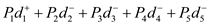Subject to,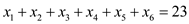Here,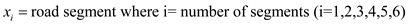Step 2: Obtain the objective function and variables in the MS excel sheet a shown below: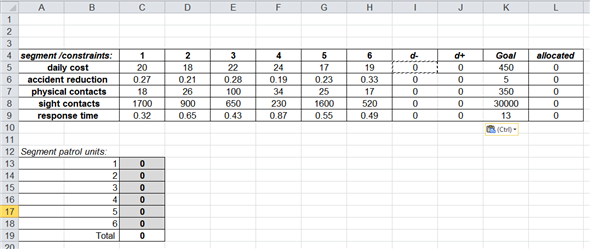Step3: Go to Data, add the requisite values and click 'Solver' as shown below: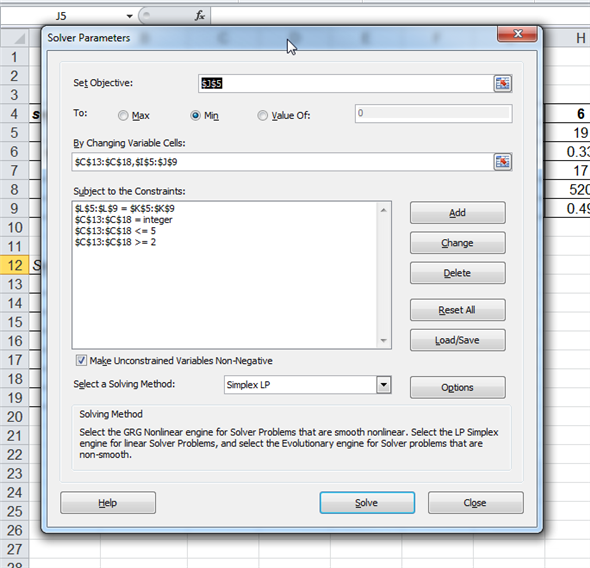Step4: The most optimal solution for the number of patrol units to assign each road segment is as follows: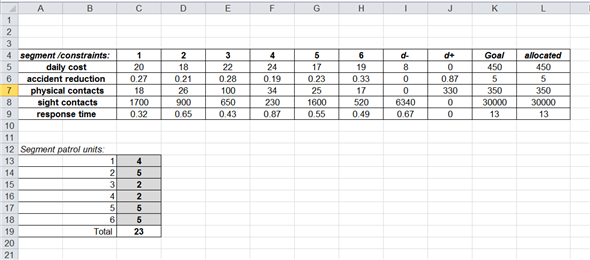Thus, the 23 patrol cars are assigned to the 6 major roads with the minimum constraints, is as follows: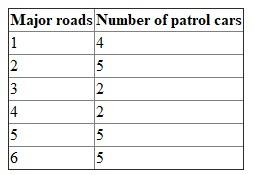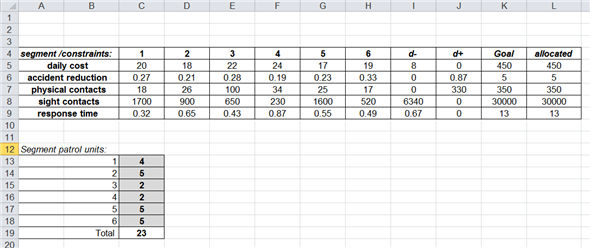Develop a ranking of the job offers for Person K, by following the step shown below: Step 1: List decision criteria's for Person K in accepting the job offers as, as shown below: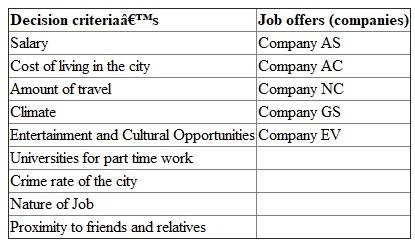These are the 9 decision criteria's for the person K to take the ranking decision regarding the job offers from the 5 companies. Step 2: Obtain weights for each of the decision criteria, as shown below :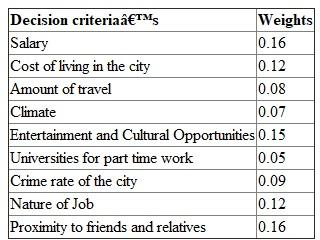Step 3: Attach grades to each of the decision criteria, in relation with company job offers, as shown below: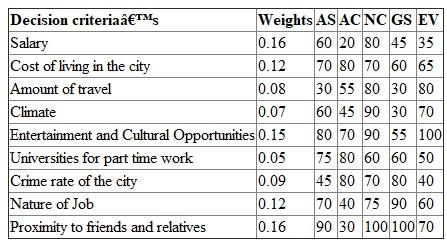Each job offer on the basis of the given decision criteria's have been attached grades with range from 0 to 100. Step 4: Calculate the scores for each restaurants to rank them from best to worst, as shown below: Calculate scores with the formula: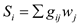Here,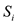= total score for decisions, i.e. higher the score, the better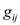=Grades between 0 to 100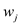= weights between 0 to 1 The scores for each job offer are calculated as shown below: S1= (0.16)(60) + (0.12)(70) + (0.08)(30) + (0.07)(60) + (0.15)(80) + (0.05)(75) + (0.09)(50) + (0.12)(70)+ (0.16)(90) = 72.15 S2 = (0.16)(20) + (0.12)(80) + (0.08)(55) + (0.07)(45) + (0.15)(70) + (0.05)(80) + (0.09)(80) + (0.12)(40) + (0.16)(30) = 69.82 S3 = (0.16) (80) + (0.12) (40) + (0.08)(80) + (0.07)(90) + (0.15)(60) + (0.05)(60) + (0.09)(50) + (0.12)(20) + (0.16)(30) = 88.71 S4 = (0.16)(45) + (0.12)(60) + (0.08)(30) + (0.07)(30) + (0.15)(55) + (0.05)(60) + (0.09)(80) + (0.12)(90) + (0.16)(100) = 68.94 S5 = (0.16)(35) + (0.12)(65) + (0.08)(80) + (0.07)(70) + (0.15)(100) + (0.05)(50) + (0.09)(40) + (0.12)(60) + (0.16)(70) = 71.23 Hus, as per the ranking list of preference of person K, job offer by Company NC is the best option and should be chosen. The ranking list of the job offer is shown as below: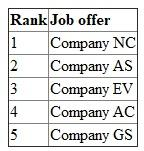Prepare the goal programing model, by following the steps as shown below: Step 1: Formulate the equations for scheduling students between the districts, as shown below: Goal: Minimize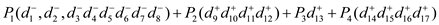Subject to: The first Priority (P1) is such that all the students (white and black) are allocated to a school which has the following 8 equations:The next Priority (P2) is to maintain 60% of white and 40% of black ratio in each school, as shown below:Priority (P3) is the goal for the mileage or the distance to be 30,000 as shown below:Here,Step 2: Obtain the objective function and variables in the MS excel sheet a shown below: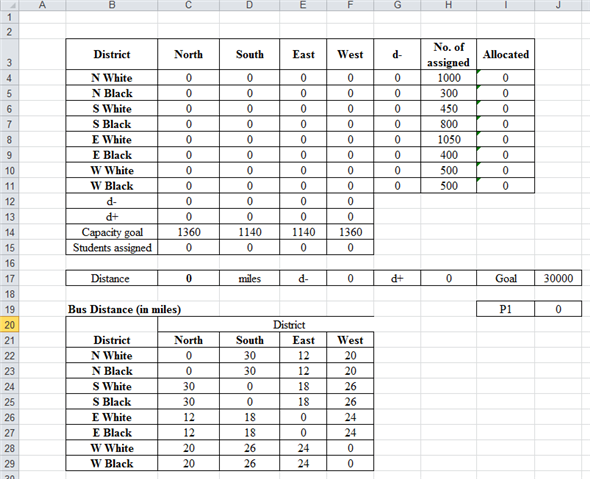Step3: Go to Data, add the requisite values and click 'Solver' as shown below: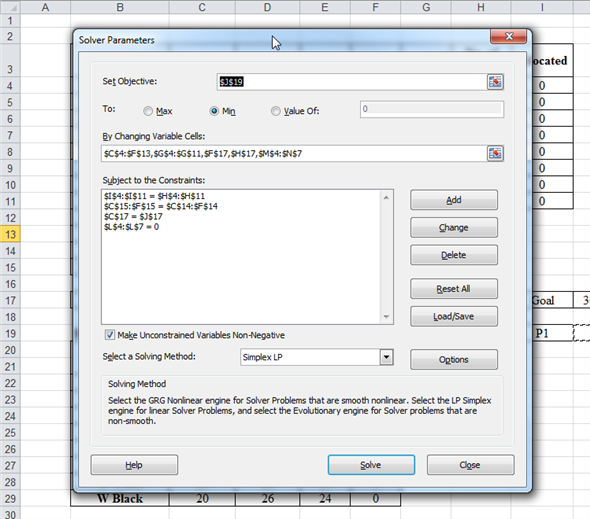Step4: The most optimal solution for the students to schedule in each district school is as follows: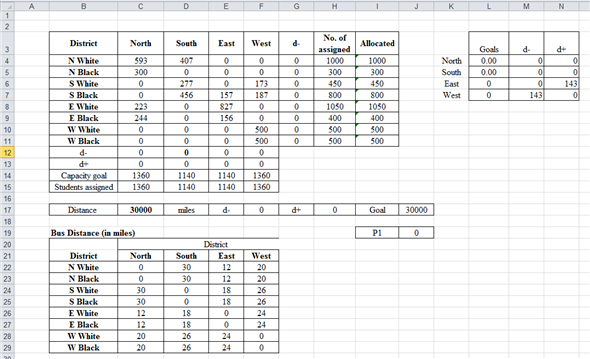Thus, the white and black students from each district to minimize the goal of allotment and distance are shown as below: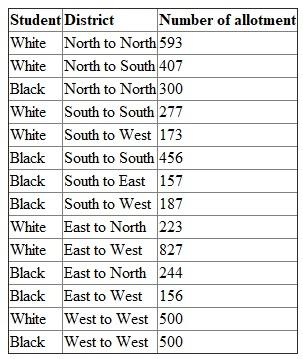There is no answer for this question

There is no answer for this question

There is no answer for this question

There is no answer for this question

There is no answer for this question

There is no answer for this question

There is no answer for this question

There is no answer for this question

There is no answer for this question

There is no answer for this question

There is no answer for this question

There is no answer for this question

There is no answer for this question

There is no answer for this question

There is no answer for this question